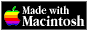THE HYPERCARD CENTER

 HyperTalk Reference
 Home » HyperTalk Reference » Operators and constants Select a destination << Home Stack < HyperTalk Reference Arithmetic operatorsComparison operatorsLogical operatorsString operatorsType and existence operatorsConstants

# Arithmetic operators

### -

Makes the number to its right negative or subtracts the number on the right from the number on the left.

```-33 3 - 2 the number of this card - 1 ```

### +

Adds the number on the left to the number on the right.

```1 + 2 the bottom of card button 1 + 5 ```

### *

Multiplies the number on the left by the number on the right.

```3 * 2 lineNumber * the textHeight of card field 1 ```

### /

Divides the number on the left by the number on the right.

```23 / 13 the number of cards / 10 the freesize of this stack / 1024 ```

### ^

Raises the number on the left to the power of the number on the right.

```3 ^ 3 2 ^ 10 2 ^ -1 10 ^ 7.1 10 ^ pi ```

### div

Divides the number on the left by the number on the right and ignores any remainder.

```100 div 13 the number of cards div 2 the freesize of this stack div 1024 ```

### mod

Divides the number on the left by the number on the right and returns the remainder.

```100 mod 19 the number of this card mod 2 ```

## Related Topics

Version 0.7b1 (March 24, 2022)Switch to Modern View